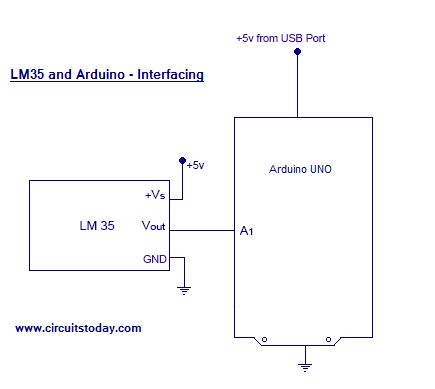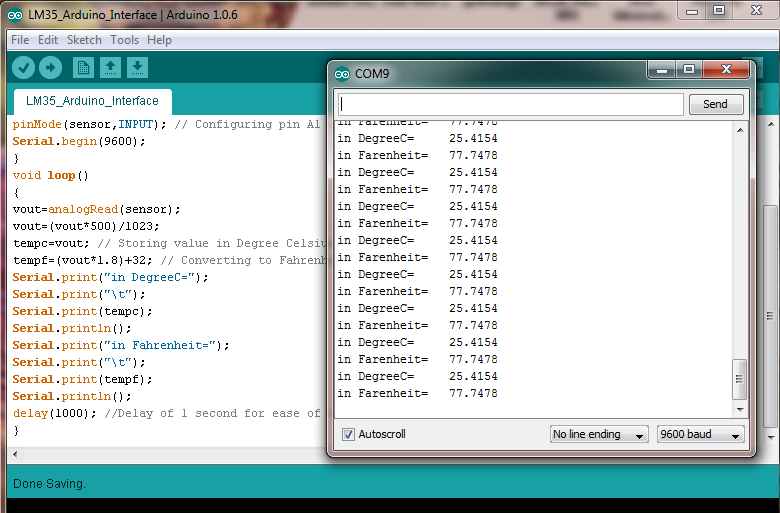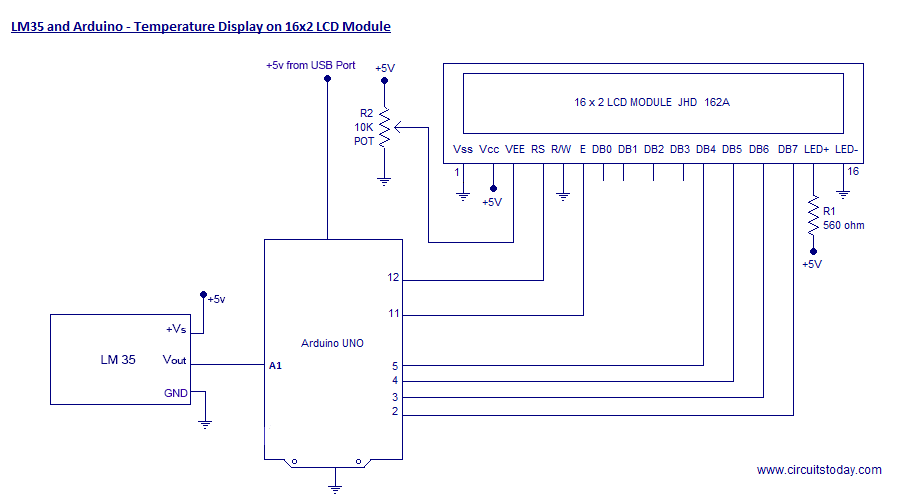## Audio

[Audio][slideshow]
In this article, we are presenting a tutorial on how to interface LM35 and Arduino along with its program. Once we successfully interface arduino and lm35, we will go on to build a temperature display using arduino and a 16×2 LCD module which constantly monitors temperature around the measurement field/range of LM35 and displays the same on LCD module. So lets get to building the project!

LM35 is an analog, linear temperature sensor whose output voltage varies linearly with change in temperature. LM35 is three terminal linear temperature sensor from National semiconductors. It can measure temperature from-55 degree celsius to +150 degree celsius. The voltage output of the LM35 increases 10mV per degree Celsius rise in temperature. LM35 can be operated from a 5V supply and the stand by current is less than 60uA. The pin out of LM35 is shown in the figure below.
So that’s all info you need about LM35 for this particular temperature display project using arduino uno. So lets get to LM35 temperature sensor interfacing with arduino!
We are using Arduino Uno as our board and LM35 can be connected to arduino as shown in circuit diagram.

Note:- LM35 is an analog temperature sensor. This means the output of LM35 is an analog signal. Microcontrollers dont accept analog signals as their input directly. We need to convert this analog output signal to digital before we can feed it to a microcontroller’s input. For this purpose, we can use an ADC( Analog to Digital Converter).If we are using a basic microcontroller like 8051, we need to use an external ADC to convert analog output from LM35 to digital. We then feed the output of ADC ( converted digital value) to input of 8051. But modern day boards like Arduino and most modern day micro controllers come with inbuilt ADC. Our arduino uno has an in built 10 bit ADC (6 channel). We can make use of this in built ADC of arduino to convert the analog output of LM35 to digital output. Since Arduino uno has a 6 channel inbuilt ADC, there are 6 analog input pins numbered from A0 to A5. Connect analog out of LM35 to any of these analog input pins of arduino.

#### LM35 and Arduino – Circuit DiagramConnect LM35 to Arduino uno as shown in circuit diagram. The +5v for LM35 can be taken from the +5v out pin of arduino uno. Also the ground pin of LM35 can be connected to GND pin of arduino uno. Connect Vout (the analog out of LM35) to any of the analog input pin of arduino uno. In this circuit diagram, we have connected Vout of LM35 to A1 of arduino.

Note:- LM35 is available in the market in 3 series variations – LM35A, LM35C and LM35D series. The main difference between these 3 versions of LM35 IC are in their range of temperature measurements. The LM35D series is designed to measure from 0 degree Celsius to 100 degree Celsius, where as the LM35A series is designed to measure a wider range of -55 degree Celsius to 155 degree Celsius. The LM35C series is designed to measure from -40 degree Celsius to 110 degree Celsius.
In our LM35 arduino example, we are using the LM35Dz sensor- which falls under LM35D series. So our min-max range of temperature measurement is 0 degree Celsius to 100 degree Celsius.
##### The Program – LM35 and Arduino Interfacing
`const int sensor=A1; // Assigning analog pin A1 to variable 'sensor'  float tempc;  //variable to store temperature in degree Celsius  float tempf;  //variable to store temperature in Fahreinheit   float vout;  //temporary variable to hold sensor reading  void setup()  {  pinMode(sensor,INPUT); // Configuring pin A1 as input  Serial.begin(9600);  }  void loop()   {  vout=analogRead(sensor);  vout=(vout*500)/1023;  tempc=vout; // Storing value in Degree Celsius  tempf=(vout*1.8)+32; // Converting to Fahrenheit   Serial.print("in DegreeC=");  Serial.print("t");  Serial.print(tempc);  Serial.println();  Serial.print("in Fahrenheit=");  Serial.print("t");  Serial.print(tempf);  Serial.println();  delay(1000); //Delay of 1 second for ease of viewing   }`
So that’s the arduino lm35 code for reading temperature and displaying in degree Celsius and Fahrenheit. The program is self explanatory.
##### The Output Screenshot of Serial MonitorOkay! We have learned the LM35 temperature sensor interfacing with arduino. If you have any doubts regarding this part, ask in comments section.

#### Temperature Display on 16×2 LCD Module – using Arduino and lM35

Now lets go on to add a 16×2 LCD display with LM35 and Arduino – interface and lets display the temperature values on this LCD display (instead of serial monitor). So we are going to build none other than a stand alone temperature display using arduino.
Note:- If you are a beginner, read our tutorial on Interfacing LCD Module to Arduino before you try your hand at this project of LM35 and Arduino with a 16×2 LCD display.
##### Circuit Diagram – LM35 and Arduino – Temperature Display on 16×2 LCDOkay! So that’s the circuit diagram to build an Arduino LM35 temperature sensor with LCD display. The arduino program for the circuit is given below.
##### The Program
`#include<LiquidCrystal.h>  LiquidCrystal lcd(12, 11, 5, 4, 3, 2);   const int sensor=A1; // Assigning analog pin A1 to variable 'sensor'  float tempc;  //variable to store temperature in degree Celsius  float tempf;  //variable to store temperature in Fahreinheit   float vout;  //temporary variable to hold sensor reading  void setup()  {  pinMode(sensor,INPUT); // Configuring pin A1 as input  Serial.begin(9600);  lcd.begin(16,2);      delay(500);  }  void loop()   {  vout=analogRead(sensor);  vout=(vout*500)/1023;  tempc=vout; // Storing value in Degree Celsius  tempf=(vout*1.8)+32; // Converting to Fahrenheit   lcd.setCursor(0,0);  lcd.print("in DegreeC= ");  lcd.print(tempc);  lcd.setCursor(0,1);  lcd.print("in Fahrenheit=");  lcd.print(tempf);  delay(1000); //Delay of 1 second for ease of viewing in serial monitor  }`
Okay! The program is very simple and self explanatory, if you have a basic idea of arduino sketches and you already learned how to interface arduino and lcd module.
Some people would like to use 7 Segment display to display temperature measured using LM35 and Arduino. so our next section explains, how to build a system using LM35 and Arduino to display temperature on a 7 segment display.
First of all, learn our tutorial on Interfacing Arduino and 7 Segment Display – which clearly explains interfacing different types of seven segment displays to arduino and how to display different numeral digits/characters on them. For displaying temperature upto 3 digit values (100 degree Celsius or higher upto 999) with corresponding unit (Celsius of Fahrenheit), its good to choose a 4 Digit 7 Segment Display unit.

[Lights][grids]